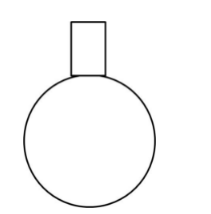# A spherical glass vessel has a cylindrical neck that is 7 cm long and 4 cm in diameter.`
Question:

A spherical glass vessel has a cylindrical neck that is 7 cm long and 4 cm in diameter. The diameter of the spherical part is 21 cm. Find the quantity of water the vessel can hold.

Solution:Diameter of spherical part = 21 cm
Radius of the spherical part = 10.5 cm

Volume of spherical part of the vessel $=\frac{4}{3} \pi r^{3}=\frac{4}{3} \times \frac{22}{7} \times 10.5 \times 10.5 \times 10.5=4851 \mathrm{~cm}^{3}$

Diameter of cylinder $=4 \mathrm{~cm}$

Radius of cylinder $=2 \mathrm{~cm}$

Height of cylinder $=7 \mathrm{~cm}$

Volume of the cylindrical part of the vessel $=\pi r^{2} h=\frac{22}{7} \times 2 \times 2 \times 7=88 \mathrm{~cm}^{3}$

Total volume of the vessel $=4851+88=4939 \mathrm{~cm}^{3}$